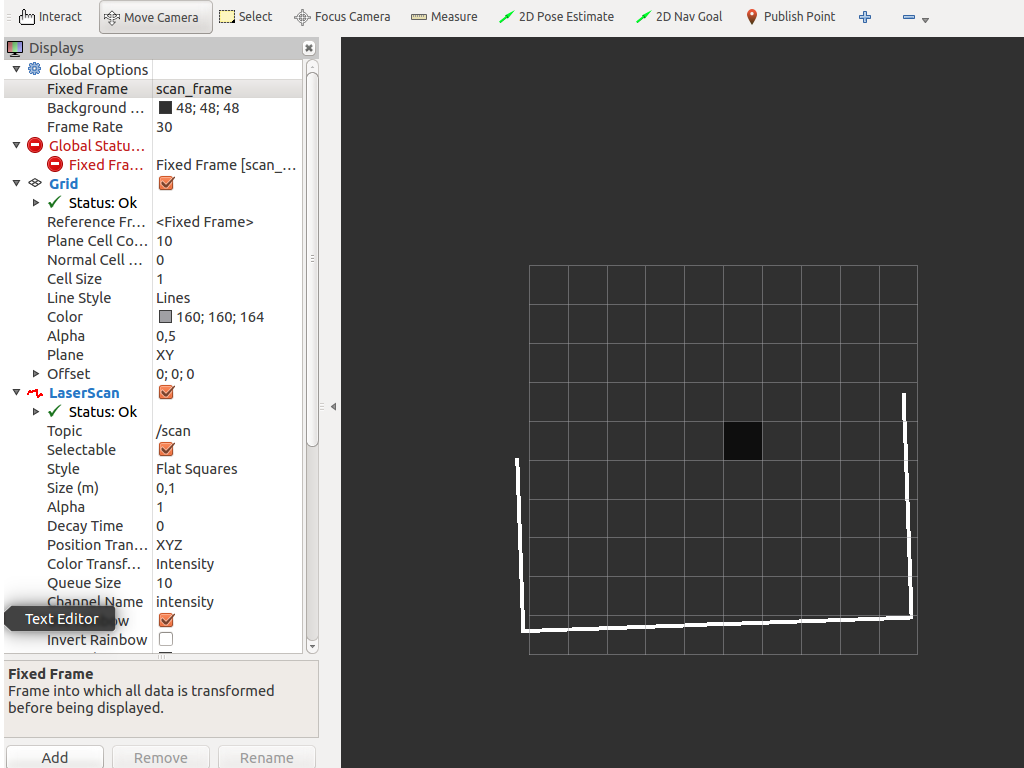# LaserScan points in laserscan frame [closed]

Hello,

i try to understand how the laserscan points are oriented in the laserscan frame. I publish my own laserscan message which includes 401 scan values in a range from -100 degrees to 100 degrees:

ls_msg.header.frame_id = "scan_frame"
ls_msg.angle_min = -100/180*math.pi
ls_msg.angle_max = 100/180*math.pi
ls_msg.angle_increment = 0.5/180*math.pi
ranges_in_mm = struct.unpack('401H',rcv_str)
ls_msg.ranges = [i/1000.0 for i in ranges_in_mm] # mm to m


But when i look at the laserscan in rviz and set the scan_frame as global fixed frame then the laserscan points start at -180 degrees.what am i doing wrong?

Thank you in advance!

EDIT:

Ok, i found my mistake. I had to make a division of float values:

ls_msg.angle_min = -100.0/180.0*math.pi
ls_msg.angle_max = 100.0/180.0*math.pi
ls_msg.angle_increment = 0.5/180.0*math.pi

edit retag reopen merge delete